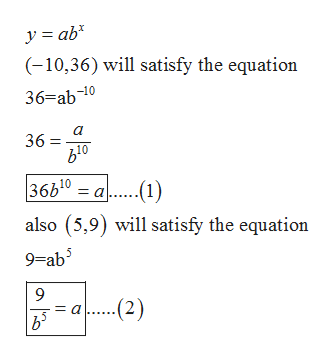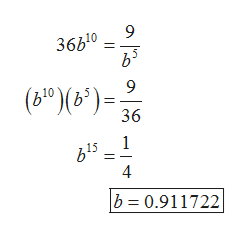Find a formula for an exponential function y=f(x)  whose graph contains the points (-10,36) and (5,9).

Question

Find a formula for an exponential function y=f(x)  whose graph contains the points (-10,36) and (5,9).

Step 1

The general form of the exponential function is:

Step 2

Since, (-10, 36) and (5, 9) lies on the graph. So it must satisfy the given function that is:help_outlineImage Transcriptionclosey = ab (-10,36) will satisfy the equation 36=ab-10 a 36 610 36b10-.1) = a also (5,9) will satisfy the equation 9=ab 9 ...2) b 5 fullscreen
Step 3

Now, solve the equation (1) and (2) to get the con...help_outlineImage Transcriptionclose9 36b10 9 (610)(6)= 36 1 4 b 0.911722 a a ON fullscreen

Want to see the full answer?

See Solution

Want to see this answer and more?

Our solutions are written by experts, many with advanced degrees, and available 24/7

See Solution
Tagged in

Other